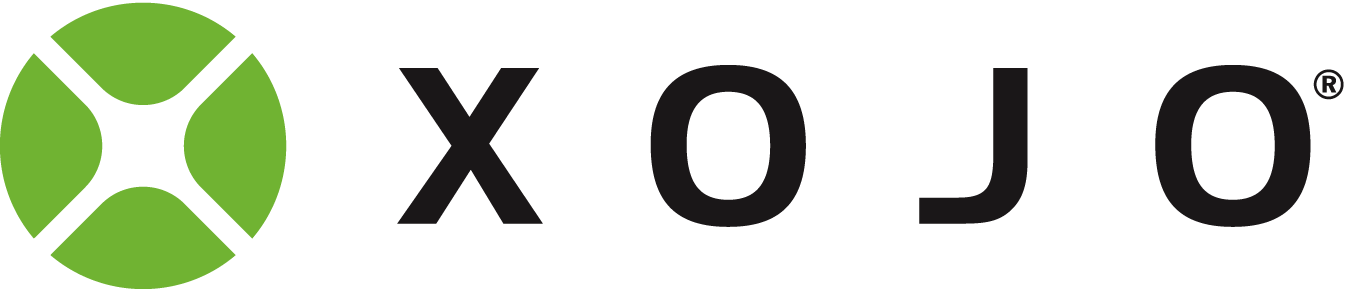# DrawPicture with Transfer Modes

Using only the “plain vanilla” methods of Xojo, is it possible to DrawPicture on a picture, using transfer modes, like Multiply, Screen, Overlay, etc?

Haven’t tried yet, but if the image is not too big (no more than 2048x2048, let’s say), using the RGBSurface property could be fast enough?

fast means 60 FPS? or seconds?

Well, I don’t need it for animation but if the calculation between two 2048x2046 RBGsurfaces could be performed in under a 1/10 of a second, I guess it would be enough.

i remember its slow (at least from debug mode) but you can test yourself.
i used this to remove a green background from a photo.

``````Public Function Alpha(red as Integer, green as Integer, blue as Integer) As Integer
'Color Difference Key

Return green-Max(red,blue)

End Function

Public Function RemoveGreen(picIn As Picture) As Picture

Var picOut As New Picture(picIn.Width,picIn.Height)
picOut.Graphics.DrawPicture(picIn,0,0)

Var surf As RGBSurface = picOut.RGBSurface

Var c As Color
For x As Integer = 0 To picIn.Width-1
For y As Integer = 0 To picIn.Height-1
c = surf.Pixel(x,y)

If Alpha(c.Red,c.Green,c.Blue) > 30  Then
surf.Pixel(x,y) = Color.Clear
Else
'surf.Pixel(x,y) = c
End If
Next
Next

Return picOut

End Function``````

Thank you so much.
I will give it a try.
Anyway, when compiled and with Agressive optimization, it should be faster, right?

1 Like

yes compiled is much faster than run from debug mode.
if you have benefit from optimization you will see.

Hello
i have some quick image warping routines
less than 1 second
an approach only with Drawpicture instead of RGBsurface

I also tried the new graphics functions
the new graphics functions are very difficult
with the drawing
I prefer my old pixmapshape elements
which I manage in an array

https://www.dropbox.com/s/l23qnivpwxv9y8o/drawpicture-Examble-fast.xojo_binary_project?dl=1

It is not possible.
As a test, I performed a Multiply transfer operation between 8 layers of 1024x800 pixels.
It took around 5 seconds.
Not fast enoughyou can only try to optimize it by static variables, var definition at top of the method, less methods calls, Pragma Directives.
but it will still use a single cpu core.
plan b would be a external command line tool for this task in c++ or using mbs plugins.

If you inline your calculations, you can save some time there.

How is that?!?Reducing the calls to functions, reduces the overheads involved in calling functions. You can also do this along the hue channel color.hue using a distance calculation to determine how far away from green it is.

Thank you. I will try that.
Also…
What is faster?
A Select Case, or IF statements, including a Continue to advance to the next iteration?

This is my code:

``````Var x,y,l As Integer
Var cor1,cor2 As Color
var r1,g1,b1,r2,g2,b2 As Double
Var final As New Picture(1024,800,32)

for y = 0 to 799
for x = 0 to 1023
cor1 = layer(layer1).RGBSurface.Pixel(x,y)
for l = layer1+1 to layer2

cor2 = layer(l).RGBSurface.Pixel(x,y)

Select Case mode
Case 1 ' Multiply
r1 = cor1.Red/255.0
g1 = cor1.Green/255.0
b1 = cor1.Blue/255.0
r2 = cor2.Red/255.0
g2 = cor2.Green/255.0
b2 = cor2.Blue/255.0
cor1 = Color.RGB(r1*r2*255,g1*g2*255,b1*b2*255)

Case 2 ' Screen
r1 = cor1.Red/255.0
g1 = cor1.Green/255.0
b1 = cor1.Blue/255.0
r2 = cor2.Red/255.0
g2 = cor2.Green/255.0
b2 = cor2.Blue/255.0
cor1 = Color.RGB((1.0-(1.0-r1)*(1.0-r2))*255,(1.0-(1.0-g1)*(1.0-g2))*255,(1.0-(1.0-b1)*(1.0-b2))*255)
end select

' otherwise, Normal transfer mode

next
final.RGBSurface.Pixel(x,y) = cor1
next
next

return final
``````

Is there any way to make it faster?

removing the /255.0 * 255
its somehow equivalent 0=0 1=255

create a integer color without .RGB

The “Multiply” calculation is usually performed with values between 0 and 1.
But, if I multiply both values (between 0 and 255) and then simply divide by 65025.
This will cut some calculations.
And, what do you mean by " integer color without .RGB"?

So, I can assign a color as an Int32?
If yes, that means that I can greatly reduce the calculations.

I can assign a color as an Int32?

the docu write this
surf.Pixel(x, y) = &cFFFFFF
https://docs.xojo.com/RGBSurface.Pixel

i guess that each method call to .RGB allocate and free memory that would be in this loop disturbing.

But, can I do something like:

surf.Pixel(x, y) = value

where value is an Int32, calculated as (red * 65536)+(green * 256)+blue

???

Forum for Xojo Programming Language and IDE. Copyright © 2021 Xojo, Inc.